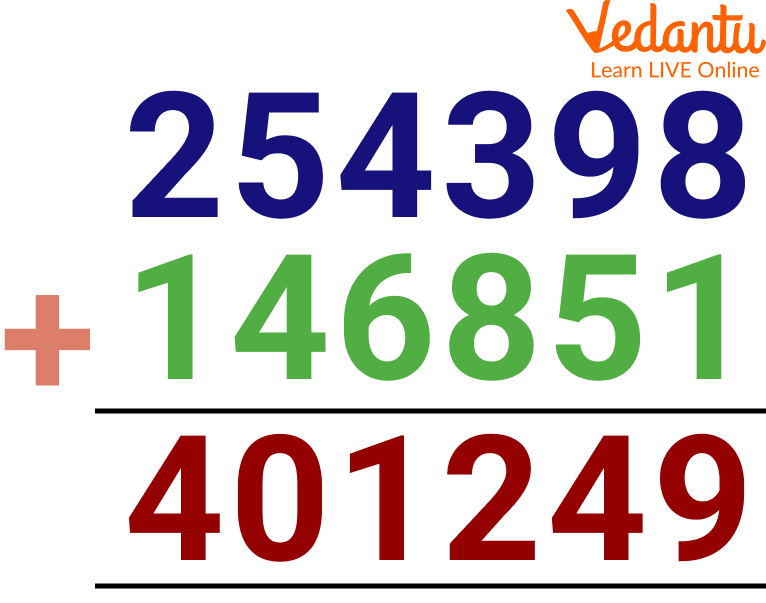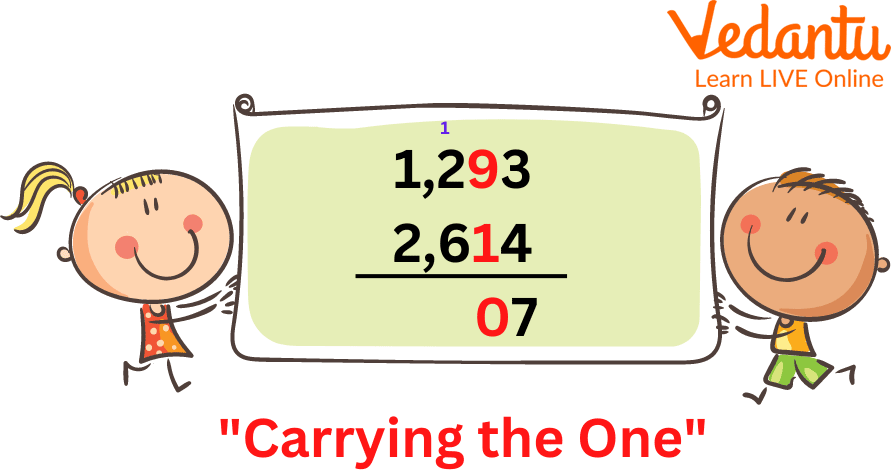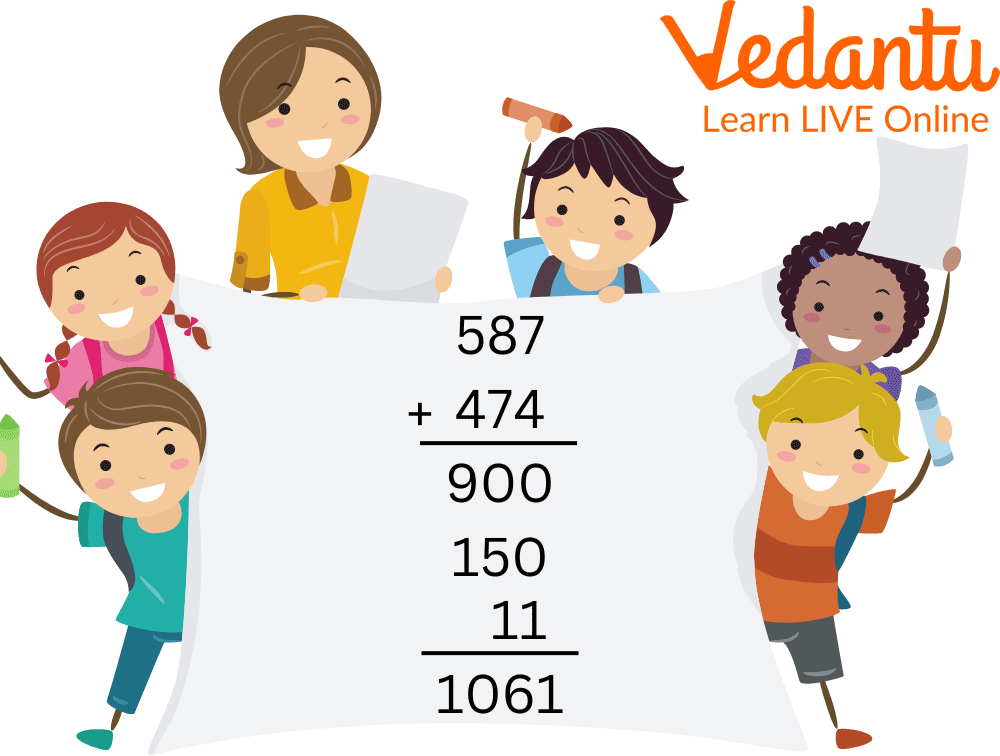Courses
Courses for Kids
Free study material
Free LIVE classes
MoreLIVE
Join Vedantu’s FREE Mastercalss

## An Overview of the Addition of Numbers

Addition is the first arithmetic calculation that we learn in the mathematical world. The addition is the basic step to moving further into the core of mathematics. In the mathematical world, addition is the way of combining things and counting all of them together as one large group. Addition can be done with any positive number, integers, and real numbers.

The symbol of addition is a plus sign (+). It is one of the four basic operations of arithmetic. To perform this type of calculation a very simple set of rules is required to follow. Further in this article, we will talk about the addition of large numbers, the addition of whole numbers, adding numbers, and what do you mean by add number.

## What is an Addition of Number?

Add number is nothing but the addition of two numbers. The addition symbol “+” is used to indicate adding two or more numbers. An add number is the addition of two unknown numbers and then getting one result. For example, 5+3 we will read this number as 5 plus 3 and the result will be 8.

Interestingly, the two or more values that are added together are called addends. Now, an additional sentence is just a mathematical expression that shows two or even more values that are added together and their sum is searched.Addition can be done using any kind of positive number in the mathematical world. One must know the method of addition, that's all. Initially, we start learning addition using small or single-digit numbers but, they can be done with larger numbers as well. But, before going to large numbers one must know these simple steps for addition.

The steps are as follows:-

• The first step is to stack the numbers according to their place value.

• The second step is to add the numbers starting from the right side of the column.

• If there are carry numbers then that will go to the left-hand side as needed. This process should be continued if there are many numbers to be calculated.

Here is an example of the addition of large numbers for the kids out there.One must start from the right-hand side while adding any number. The first step here is 8+1=9. Then there is 5+9=14 so you will need to write 4 below and 1 will carry forward to the next column for addition. Followed by 8+3=11+1=12, we need to write 2 below and 1 will carry forward to the next column. There is 4+6=10+1=11, 1 will be written below and 1 will be carried forward to the next column. There is 4+5=9+1=10, 0 will be written below and 1 will be carried forward to the next column. The last column is 2+1=3+1=4, which will be written below.Carry Over While Adding Two Numbers

Before making any addition one must know what are whole numbers. Whole numbers are all the numbers but they start from 0. It is all the counting numbers that we use in daily lives but including 0.

Any number used for addition is a whole number. For example, 0+2=2 is an addition done by using two whole numbers. Another example of the addition of whole numbers (115 + 37 = 152) is a great example of the addition of whole numbers. There are some great examples of adding numbers for kids to understand.

Now, we are quite sure kids will be able to solve addition with whole numbers and large numbers. But, there is a correct saying “Practice makes a man perfect” which is quite accurate. Mathematics is such a subject that not only requires understanding but, it requires a lot of practice to become better at it.

By now the little ones must develop a love for maths by understanding them and solving tricky questions and sums. The more the difficulty the more interesting it becomes for the little ones out there. Here are some big addition sums along with their answers just write in the column and add:-

1. 72956 and 62450= 135406

2. 198765, 500321, and 4056= 703142

3. 980, 280, 480, and 112= 1852

4. 56879 and 63275= 120154

These are some examples of big addition sums for kids to try on their own with other numbers after understanding.Here we have added numbers according to their place values, for example from 587 we took 500 and from 474 we took 400 for hundreds position then added together. Similarly for tens position we took 80 and 70 and for ones position we added 7 and 4. Then we added all these together to get the sum.

## Solved Examples

Ques 1. Add 4372 and 2125.

Ans. Write the digits in column form, then start adding the numbers from the right side then move to the left side. Add all the digits one by one and write their sum corresponding to the digits.

Thus the addition of 4372 and 2125 is 6497.

Ques 2. Solve: 34246+3523

Ans. Write the digits in column form, then start adding the numbers from the right side then move to the left side. Add all the digits one by one and write their sum corresponding to the digits.

Thus the addition of 34246 and 3523 is 37769.

## Practice Questions

Solve the following big addition sums:

Q 1. 525 + 25 + 40

Ans: 590

Q 2. 19 + 52 + 61

Ans: 132

Q 3. 637 + 400

Ans: 1037

Q 4. 968 + 875 + 682

Ans: 2525

Q 5. 533348 + 366992

Ans: 900340

Q 6. 621239 + 155632 + 119215

Ans: 896086

## Summary

The addition is the basic form of mathematics. There are numerous activities where a child can learn addition at school and home. If the basics are taught and learned well then there is no falling back for the little ones. Moreover, we are quite sure that the kids now have a little idea of how to do addition and what are their processes. In this article, we have learned about what add numbers are, the addition of numbers, which are whole and large, and big addition sums.

Last updated date: 26th Sep 2023
Total views: 94.5k
Views today: 0.94k

## FAQs on Addition of Numbers

1. What are the names of three parts in an addition sum?

The three main parts of an addition sum are the addend, the equal sign, and the sum itself.

2. What are the two terms that are highly used while doing an addition?

The two terms that are mostly used while the addition is addends, and the total value is called the sum.

3. What are the four properties in addition?

The four different properties in addition are the following:-

• Commutative Property

• Associative Property

• Distributive Property